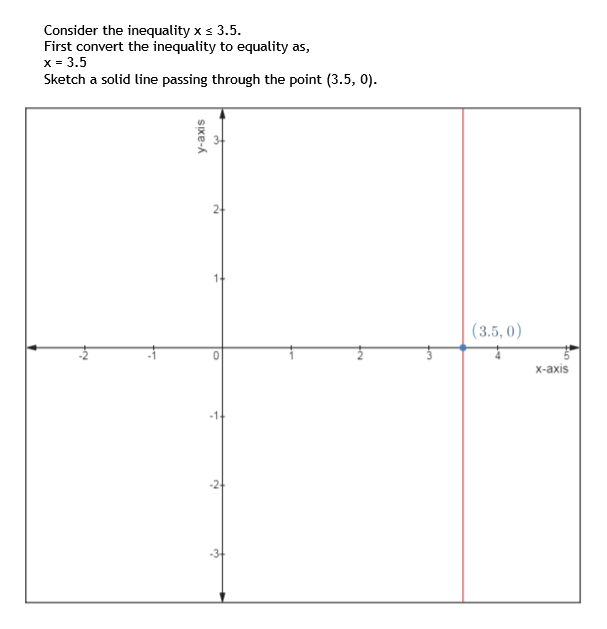Question
8 views

For Exercise, graph the solution set.

x ≤ 3.5

check_circle

Step 1

To determine the solution set of the following inequality.

Step 2

Given:

Step 3

Consider the inequality x ≤ 3.5.

First convert the inequality to equality as,

x ...help_outlineImage TranscriptioncloseConsider the inequality x s 3.5. First convert the inequality to equality as, x = 3.5 Sketch a solid line passing through the point (3.5, 0). (3.5, 0) x-axis 24 Sxe-A fullscreen

### Want to see the full answer?

See Solution

#### Want to see this answer and more?

Solutions are written by subject experts who are available 24/7. Questions are typically answered within 1 hour.*

See Solution
*Response times may vary by subject and question.
Tagged in

### Equations and In-equations